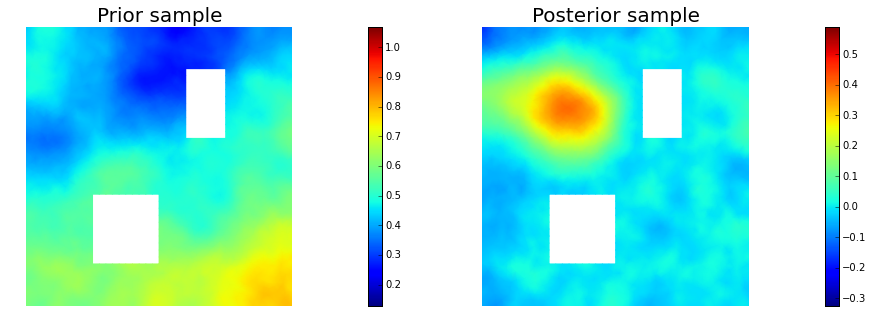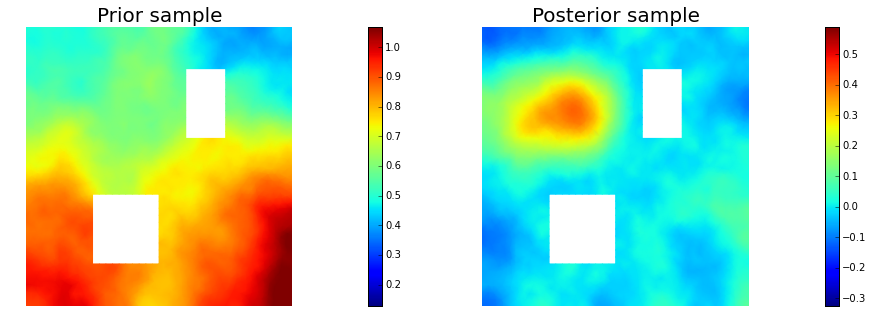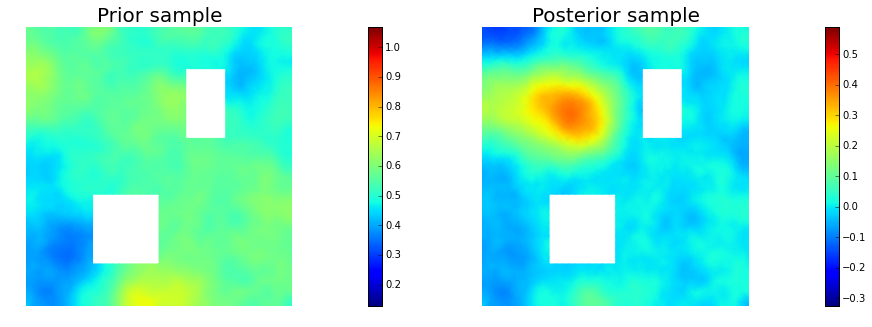$\def\D{\mathcal{D}} \def\ipar{m} \def\R{\mathbb{R}} \def\del{\partial} \def\vec{\bf} \def\priorm{\mu_0} \def\C{\mathcal{C}} \def\Acal{\mathcal{A}} \def\postm{\mu_{\rm{post}}} \def\iparpost{\ipar_\text{post}} \def\obs{\vec{d}} \def\yobs{\obs^{\text{obs}}} \def\obsop{\mathcal{B}} \def\dd{\vec{\bar{d}}} \def\iFF{\mathcal{F}} \def\iFFadj{\mathcal{F}^*} \def\ncov{\Gamma_{\mathrm{noise}}}$

# Example: Bayesian initial condition inversion in an advection-diffusion problem

In this example we tackle the problem of quantifying the uncertainty in the solution of an inverse problem governed by a parabolic PDE via the Bayesian inference framework. The underlying PDE is a time-dependent advection-diffusion equation in which we seek to infer an unknown initial condition from spatio-temporal point measurements.

### The Bayesian inverse problem:

Following the Bayesian framework, we utilize a Gaussian prior measure $\priorm = \mathcal{N}(\ipar_0,\C_0)$, with $\C_0=\Acal^{-2}$ where $\Acal$ is an elliptic differential operator as described in the PoissonBayesian example, and use an additive Gaussian noise model. Therefore, the solution of the Bayesian inverse problem is the posterior measure, $\postm = \mathcal{N}(\iparpost,\C_\text{post})$ with $\iparpost$ and $\C_\text{post}$.

• The posterior mean $\iparpost$ is characterized as the minimizer of

which can also be interpreted as the regularized functional to be minimized in deterministic inversion. The observation operator $\mathcal{B}$ extracts the values of the forward solution $u$ on a set of locations $\{\vec{x}_1, \ldots, \vec{x}_n\} \subset \D$ at times $\{t_1, \ldots, t_N\} \subset [0, T]$.

• The posterior covariance $\C_{\text{post}}$ is the inverse of the Hessian of $\mathcal{J}(\ipar)$, i.e.,

### The forward problem:

The PDE in the parameter-to-observable map $\iFF$ models diffusive transport in a domain $\D \subset \R^d$ ($d \in \{2, 3\}$):

Here, $\kappa > 0$ is the diffusion coefficient and $T > 0$ is the final time. The velocity field $\vec{v}$ is computed by solving the following steady-state Navier-Stokes equation with the side walls driving the flow:

Here, $q$ is pressure, $\text{Re}$ is the Reynolds number. The Dirichlet boundary data $\vec{g} \in \R^d$ is given by $\vec{g} = \vec{e}_2$ on the left wall of the domain, $\vec{g}=-\vec{e}_2$ on the right wall, and $\vec{g} = \vec{0}$ everywhere else.

from __future__ import absolute_import, division, print_function

import dolfin as dl
import math
import numpy as np
import matplotlib.pyplot as plt
%matplotlib inline
import sys
import os
sys.path.append( os.environ.get('HIPPYLIB_BASE_DIR', "../") )
from hippylib import *
sys.path.append( os.environ.get('HIPPYLIB_BASE_DIR', "..") + "/applications/ad_diff/" )

import nb

import logging
logging.getLogger('FFC').setLevel(logging.WARNING)
logging.getLogger('UFL').setLevel(logging.WARNING)
dl.set_log_active(False)

np.random.seed(1)


## 2. Construct the velocity field

def v_boundary(x,on_boundary):
return on_boundary

def q_boundary(x,on_boundary):
return x < dl.DOLFIN_EPS and x < dl.DOLFIN_EPS

def computeVelocityField(mesh):
Xh = dl.VectorFunctionSpace(mesh,'Lagrange', 2)
Wh = dl.FunctionSpace(mesh, 'Lagrange', 1)
if dlversion() <= (1,6,0):
XW = dl.MixedFunctionSpace([Xh, Wh])
else:
mixed_element = dl.MixedElement([Xh.ufl_element(), Wh.ufl_element()])
XW = dl.FunctionSpace(mesh, mixed_element)

Re = 1e2

g = dl.Expression(('0.0','(x < 1e-14) - (x > 1 - 1e-14)'), degree=1)
bc1 = dl.DirichletBC(XW.sub(0), g, v_boundary)
bc2 = dl.DirichletBC(XW.sub(1), dl.Constant(0), q_boundary, 'pointwise')
bcs = [bc1, bc2]

vq = dl.Function(XW)
(v,q) = dl.split(vq)
(v_test, q_test) = dl.TestFunctions (XW)

def strain(v):

F = ( (2./Re)*dl.inner(strain(v),strain(v_test))+ dl.inner (dl.nabla_grad(v)*v, v_test)
- (q * dl.div(v_test)) + ( dl.div(v) * q_test) ) * dl.dx

dl.solve(F == 0, vq, bcs, solver_parameters={"newton_solver":
{"relative_tolerance":1e-4, "maximum_iterations":100}})

plt.figure(figsize=(15,5))
vh = dl.project(v,Xh)
qh = dl.project(q,Wh)
nb.plot(nb.coarsen_v(vh), subplot_loc=121,mytitle="Velocity")
nb.plot(qh, subplot_loc=122,mytitle="Pressure")
plt.show()

return v


## 3. Set up the mesh and finite element spaces

mesh = dl.refine( dl.Mesh("ad_20.xml") )
wind_velocity = computeVelocityField(mesh)
Vh = dl.FunctionSpace(mesh, "Lagrange", 1)
print("Number of dofs: {0}".format( Vh.dim() ) )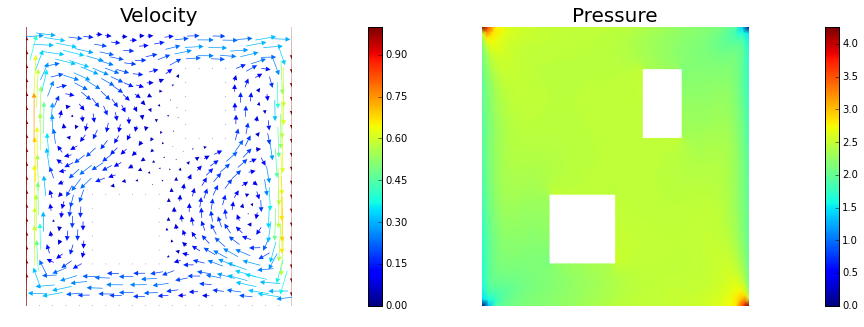Number of dofs: 2023


## 4. Set up model (prior, true/proposed initial condition)

#gamma = 1
#delta = 10
#prior = LaplacianPrior(Vh, gamma, delta)

gamma = 1
delta = 8
prior = BiLaplacianPrior(Vh, gamma, delta)

prior.mean = dl.interpolate(dl.Constant(0.5), Vh).vector()
true_initial_condition = dl.interpolate(dl.Expression('min(0.5,exp(-100*(pow(x-0.35,2) +  pow(x-0.7,2))))', degree=5), Vh).vector()
problem = TimeDependentAD(mesh, [Vh,Vh,Vh], 0., 4., 1., .2, wind_velocity, True, prior)

objs = [dl.Function(Vh,true_initial_condition),
dl.Function(Vh,prior.mean)]
mytitles = ["True Initial Condition", "Prior mean"]
nb.multi1_plot(objs, mytitles)
plt.show()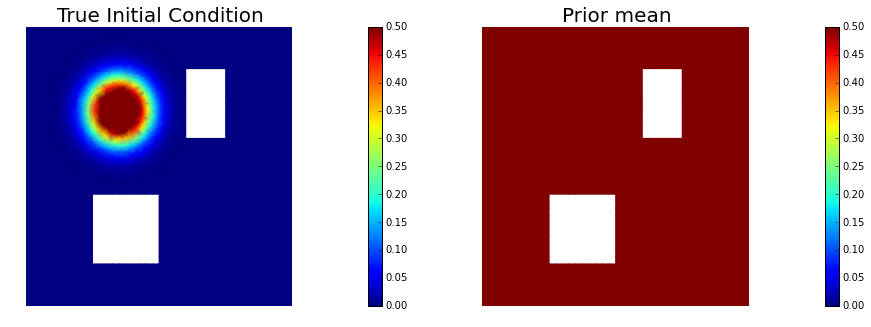## 5. Generate the synthetic observations

rel_noise = 0.001
utrue = problem.generate_vector(STATE)
x = [utrue, true_initial_condition, None]
problem.solveFwd(x[STATE], x, 1e-9)
MAX = utrue.norm("linf", "linf")
noise_std_dev = rel_noise * MAX
problem.ud.copy(utrue)
problem.ud.randn_perturb(noise_std_dev)
problem.noise_variance = noise_std_dev*noise_std_dev

nb.show_solution(Vh, true_initial_condition, utrue, "Solution")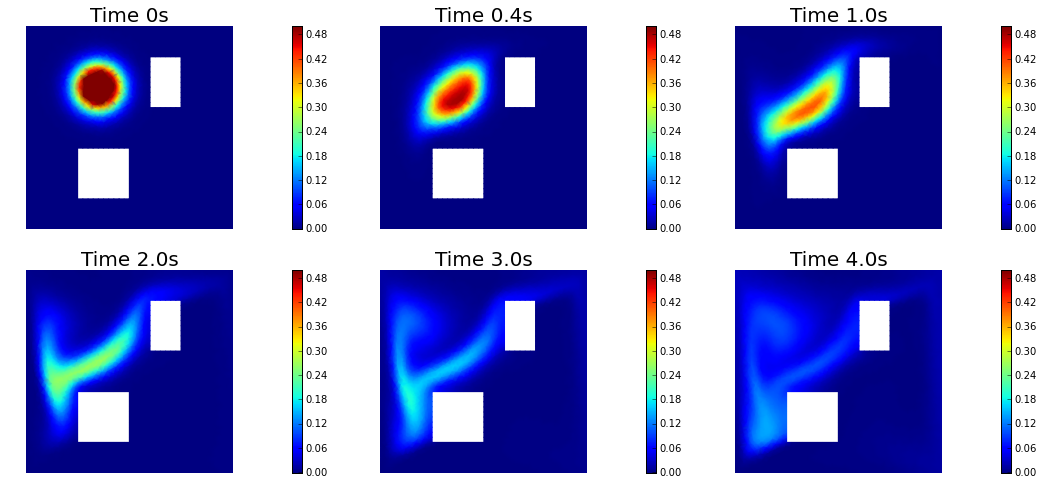## 6. Test the gradient and the Hessian of the cost (negative log posterior)

a0 = true_initial_condition.copy()

(yy, H xx) - (xx, H yy) =  -4.75341826113e-14[u,a,p] = problem.generate_vector()
problem.solveFwd(u, [u,a,p], 1e-12)
mg = problem.generate_vector(PARAMETER)


(g,g) =  1.66716039169e+12


## 8. The Gaussian approximation of the posterior

H = ReducedHessian(problem, 1e-12, gauss_newton_approx=False, misfit_only=True)

k = 80
p = 20
print("Single Pass Algorithm. Requested eigenvectors: {0}; Oversampling {1}.".format(k,p))
Omega = np.random.randn(get_local_size(a), k+p)
d, U = singlePassG(H, prior.R, prior.Rsolver, Omega, k)

posterior = GaussianLRPosterior( prior, d, U )

plt.plot(range(0,k), d, 'b*', range(0,k+1), np.ones(k+1), '-r')
plt.yscale('log')
plt.xlabel('number')
plt.ylabel('eigenvalue')

nb.plot_eigenvectors(Vh, U, mytitle="Eigenvector", which=[0,1,2,5,10,20,30,45,60])

Single Pass Algorithm. Requested eigenvectors: 80; Oversampling 20.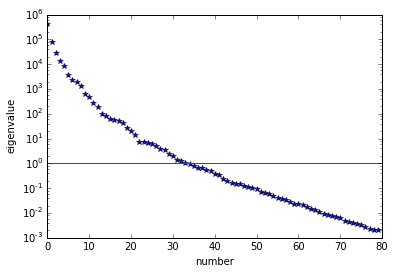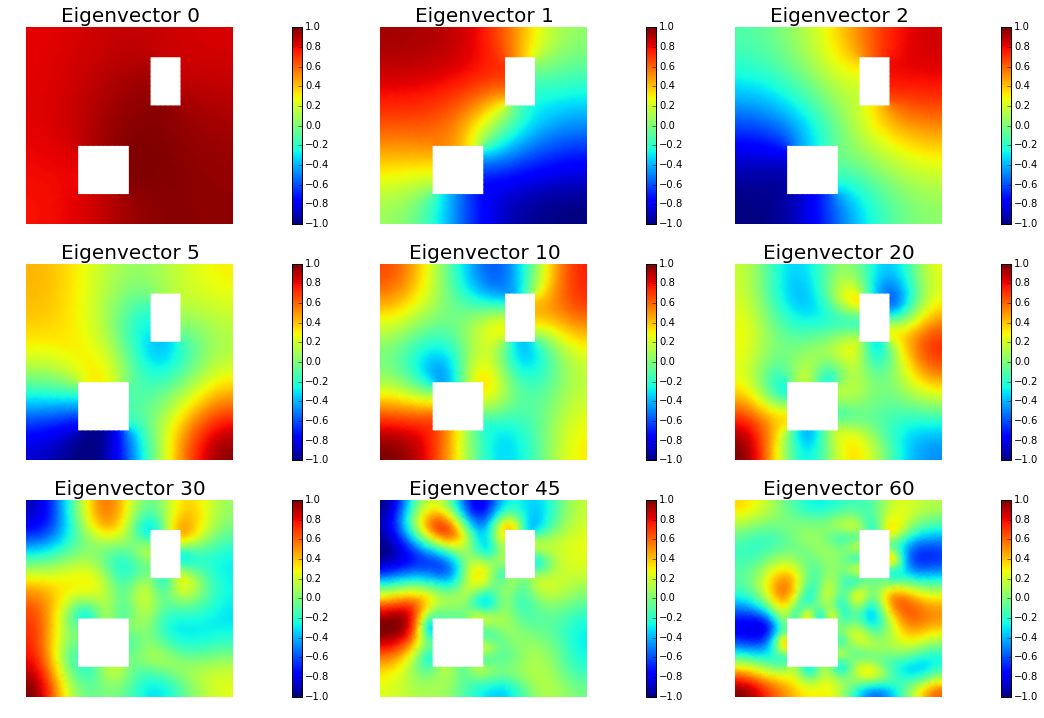## 9. Compute the MAP point

H.misfit_only = False

solver = CGSolverSteihaug()
solver.set_operator(H)
solver.set_preconditioner( posterior.Hlr )
solver.parameters["print_level"] = 1
solver.parameters["rel_tolerance"] = 1e-6
solver.solve(a, -mg)
problem.solveFwd(u, [u,a,p], 1e-12)

total_cost, reg_cost, misfit_cost = problem.cost([u,a,p])
print("Total cost {0:5g}; Reg Cost {1:5g}; Misfit {2:5g}".format(total_cost, reg_cost, misfit_cost))

posterior.mean = a

plt.figure(figsize=(7.5,5))
nb.plot(dl.Function(Vh, a), mytitle="Initial Condition")
plt.show()

nb.show_solution(Vh, a, u, "Solution")

 Iterartion :  0  (B r, r) =  30140.7469425
Iteration :  1  (B r, r) =  0.0653733954192
Iteration :  2  (B r, r) =  6.28002367536e-06
Iteration :  3  (B r, r) =  9.57007003125e-10
Relative/Absolute residual less than tol
Converged in  3  iterations with final norm  3.09355297858e-05
Total cost 84.2612; Reg Cost 68.8823; Misfit 15.3789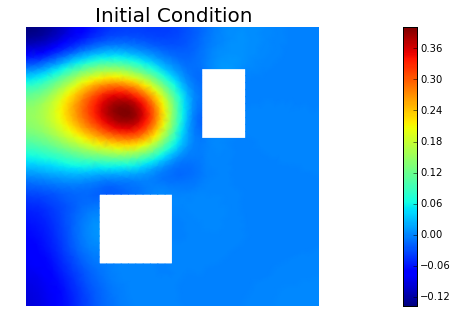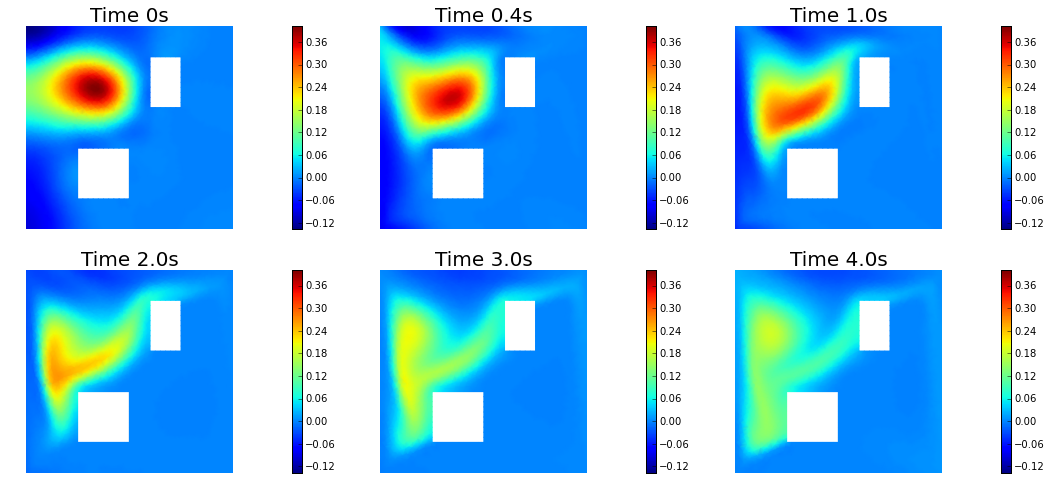## 10. Prior and posterior pointwise variance fields

compute_trace = True
if compute_trace:
post_tr, prior_tr, corr_tr = posterior.trace(method="Randomized", r=200)
print("Posterior trace {0:5g}; Prior trace {1:5g}; Correction trace {2:5g}".format(post_tr, prior_tr, corr_tr))
post_pw_variance, pr_pw_variance, corr_pw_variance = posterior.pointwise_variance(method="Randomized", r=300)

objs = [dl.Function(Vh, pr_pw_variance),
dl.Function(Vh, post_pw_variance)]
mytitles = ["Prior Variance", "Posterior Variance"]
nb.multi1_plot(objs, mytitles, logscale=True)
plt.show()

Posterior trace 0.000602854; Prior trace 0.0285673; Correction trace 0.0279644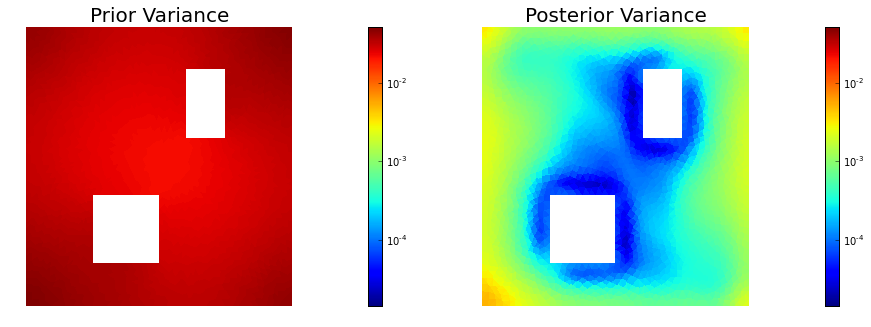## 11. Draw samples from the prior and posterior distributions

nsamples = 5
noise = dl.Vector()
posterior.init_vector(noise,"noise")
noise_size = get_local_size(noise)
s_prior = dl.Function(Vh, name="sample_prior")
s_post = dl.Function(Vh, name="sample_post")

pr_max =  2.5*math.sqrt( pr_pw_variance.max() ) + prior.mean.max()
pr_min = -2.5*math.sqrt( pr_pw_variance.min() ) + prior.mean.min()
ps_max =  2.5*math.sqrt( post_pw_variance.max() ) + posterior.mean.max()
ps_min = -2.5*math.sqrt( post_pw_variance.max() ) + posterior.mean.min()

for i in range(nsamples):
noise.set_local( np.random.randn( noise_size ) )
posterior.sample(noise, s_prior.vector(), s_post.vector())
plt.figure(figsize=(15,5))
nb.plot(s_prior, subplot_loc=121,mytitle="Prior sample", vmin=pr_min, vmax=pr_max)
nb.plot(s_post, subplot_loc=122,mytitle="Posterior sample", vmin=ps_min, vmax=ps_max)
plt.show()